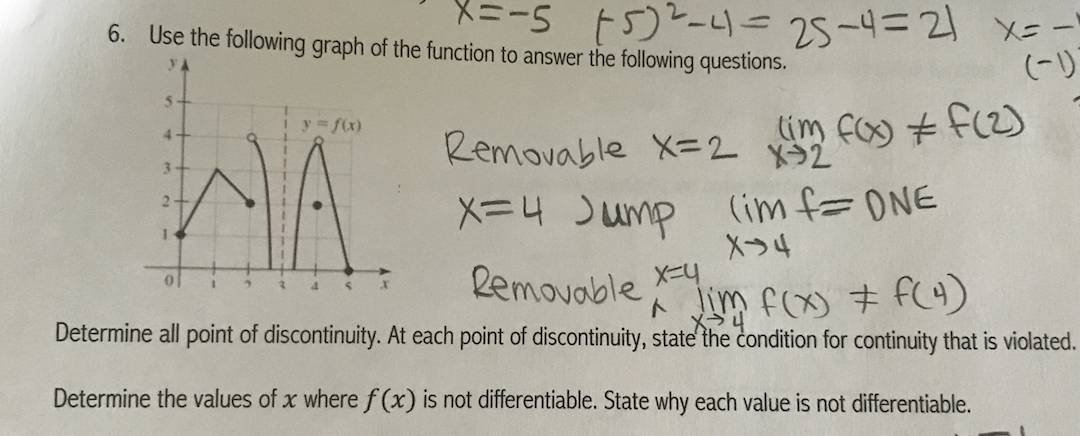# 6.Use the following graph of the function to answer the following questions.(Dy = f(x)Removable X2 24uMp (im fONERemovoble4Determine all point of discontinuity. At each point of discontinuity, state the condition for continuity that is violated.Determine the values of x where f(x) is not differentiable. State why each value is not differentiable.

Question
3 views

please answer the two questions that are part of 6's graph. thank youhelp_outlineImage Transcriptionclose6. Use the following graph of the function to answer the following questions. (D y = f(x) Removable X2 2 4uMp (im fONE Removoble 4 Determine all point of discontinuity. At each point of discontinuity, state the condition for continuity that is violated. Determine the values of x where f(x) is not differentiable. State why each value is not differentiable. fullscreen
check_circle

Step 1

To discuss the continuity and diiferentiablity of the  function (from the graph)

Step 2

f(x) has a jump discontinuity at x=2, It is not different...

### Want to see the full answer?

See Solution

#### Want to see this answer and more?

Solutions are written by subject experts who are available 24/7. Questions are typically answered within 1 hour.*

See Solution
*Response times may vary by subject and question.
Tagged in

### Derivative